### IMO Shortlist 2001 problem N2

Kvaliteta:
Avg: 0.0
Težina:
Avg: 6.0
Consider the system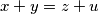$x + y = z + u$,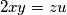$2xy = zu$. Find the greatest value of the real constant$m$ such that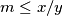$m \leq x/y$ for any positive integer solution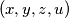$(x,y,z,u)$ of the system, with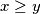$x \geq y$.
Source: Međunarodna matematička olimpijada, shortlist 2001